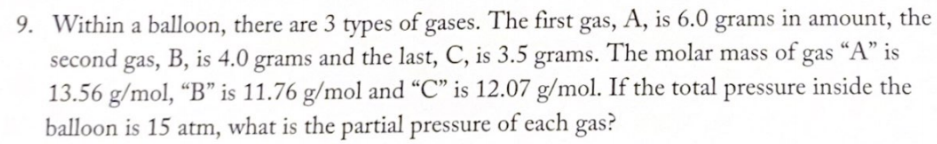# 9. Within a balloon, there are 3 types of gases. The first gas, A is 6.0 grams in amount, the second gas, B, is 4.0 grams and the last, C, is 3.5 grams. The molar mass of gas "A" is 13.56 g/mol, "B" is 11.76 g/mol and "C" is 12.07 g/mol. If the total pressure inside the balloon is 15 atm, what is the partial pressure of each gas?